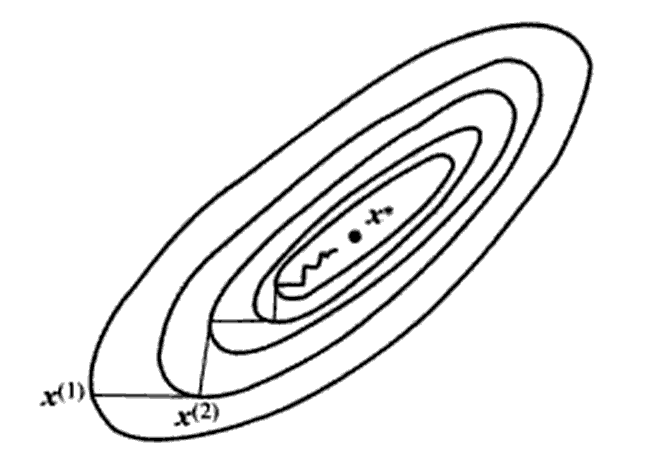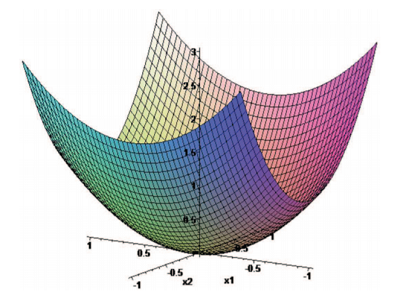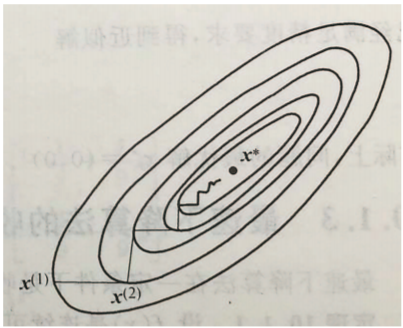• 最速下降法PPT及MATLAB程序;最速下降法的基本思想;给定x0>0k=0;例. 用最速下降法求函数f (x1, x2)2x12+x22 的极小点,取x0=[1,1]T , =0.1;得;得;得;最速下降法的搜索路径呈直角锯齿形;改进后的算法
• ## 最速下降法/梯度下降法

万次阅读 多人点赞 2017-11-03 17:20:30
锯齿现象梯度下降法在机器学习中是经常用到一种方法，很多人也把梯度下降法看作是最速下降法，但是这两种方法好像还有一些细微差别，wikipedia中Gradient descent描述中有这么一句：Gradient descent is also ...


基本思想
算法描述
应用于正定二次函数
锯齿现象

梯度下降法在机器学习中是经常用到的一种方法，很多人也把梯度下降法看作是最速下降法，但是这两种方法好像还有一些细微差别，wikipedia中Gradient descent的描述中有这么一句：Gradient descent is also known as steepest descent. However, gradient descent should not be confused with the method of steepest descent for approximating integrals.由于我也没有弄明白梯度下降和最速下降的区别，所以本文中将会用最速下降来统一说明。
在讲解梯度下降算法之前，先讲一下梯度下降的解决的问题是什么。梯度下降解决的是无约束最优化问题，与之相对应的是约束最优化问题。无约束最优化问题的一般形式为：minf(x)min\quad f(x)其中f:Rn→R1$f:R^n \to R^1$。这一问题是指在Rn$R^n$中寻找一点x∗$x^*$，使得对于∀x∈Rn$\forall x \in R^n$，都与f(x∗)≤f(x)f(x^*)\le f(x)点x∗$x^*$就是全局最优解。
梯度下降法是多元函数求极值最早的方法。梯度下降法简单直观，但是收敛速度慢。之所以收敛速度慢是由于梯度下降会出现锯齿现象，将会慢慢讲解。

基本思想

最速下降法通过迭代的方式求函数f(x)$f(x)$的最优解。给定一个初始点，通过迭代找到下一个点，我们希望找到的下一个点能比上一个点有更优的函数值。那么最速下降法最重要的一点就是应该如何迭代，这用到了上一篇博客中的一个小知识：给定点xk$x_k$，点xk$x_k$处的负梯度方向为最速下降方向，至少在点xk$x_k$的临近范围内是这样的。所以我们可以在点xk$x_k$处选择搜索方向pk=−∇f(xk)。p_k=-\nabla f(x_k)。在选定了搜索方向之后我们就可以沿着搜索方向进行搜索，然后选择点xk+1$x_{k+1}$，其中xk+1=xk−tk∇f(xk)x_{k+1}=x_k-t_k\nabla f(x_k)，其中tk$t_k$为沿负梯度方向的搜索距离，我们称为步长因子。我们把上式成为最速下降迭代公式，可以简记为xk+1=1s(xk,−∇f(xk))x_{k+1}=1s(x_k,-\nabla f(x_k))，由该公式产生的算法称为最速下降法。
在知道迭代公式之后，我们希望得到步长因子tk$t_k$能够满足f(xk−tk∇f(xk))=minf(xk−t∇f(xk))f(x_k-t_k\nabla f(x_k))=min f(x_k-t\nabla f(x_k)) ，即我们希望xk+1$x_{k+1}$为搜索方向上函数值“最小”的点。
对于任意给定的函数f(x)$f(x)$，最速下降法不一定能找到函数的全局最优点（全局极小点），可能会找找到函数的局部极小点。

算法描述

为了书写简单，记gk=g(xk)=∇f(xk)$g_k=g(x_k)=\nabla f(x_k)$。
最速下降法
已知：目标函数f(x)$f(x)$以及梯度g(x)$g(x)$，H终止准则所需要的终止限ϵ1，ϵ2，ϵ3$\epsilon_1，\epsilon_2，\epsilon_3$

1:选择初始点x0$x_0$；计算f0=f(x0)$f_0= f(x_0)$，g0=g(x0)$g_0=g(x_0)$；置k=0$k=0$；
2:作直线搜索xk+1=1s(xk,−gk)$x_{k+1}=1s(x_k, -g_k)$；计算fk+1=f(xk+1)$f_{k+1}=f(x_{k+1})$，gk+1=g(xk+1)$g_{k+1}=g(x_{k+1})$；
3:判断H终止准则是否满足：若满足，则输出xk+1$x_{k+1}$，fk+1$f_{k+1}$否则，置k=k+1$k=k+1$，转2。

关于直线搜索和H终止准则我将会在在下一篇博客中做补充说明。直线搜索是为了求解一元函数极小化问题而的迭代方法（在算法描述中使用直线搜索来找最优的tk$t_{k}$，从而通过公式xk+1=xk−tk∇f(xk)$x_{k+1}=x_k-t_k\nabla f(x_k)$找到下一个迭代点xk+1$x_{k+1}$，需要知道的是在这里求解tk$t_k$时，并一定使用迭代法，在一元函数可微或者可导的情况下，也可以直接通过导数方法进行求解），在这里是为了求得每一步的步长因子tk$t_k$；H终止准则是一种终止条件，常见的终止条件如函数值的变化范围小于阈值ϵ$\epsilon$时终止。

应用于正定二次函数

在介绍了最速下降法的基本逻辑之后，我们可以知道最速下降法关键步骤是求解每一步的步长因子tk$t_k$，而对于正定二次函数f(x)=12xTQx+bTx+cf(x)=\frac 12x^TQx+b^Tx+c是可以推出显示迭代公式的。推导过程如下。
设第k$k$个迭代点为xk$x_k$，求xk+1$x_{k+1}$的表达式。正定二次函数f(x)$f(x)$的一阶偏导数为g(x)=Qx+b$g(x)=Qx+b$，因此gk=g(xk)=Qxk+b$g_k=g(x_k)=Qx_k+b$。从xk$x_k$出发做直线搜索便可以得到xk+1$x_{k+1}$，即xk+1=xk−tkgk$x_{k+1}=x_k-t_kg_k$，tk$t_k$为最优步长因子。
由于最速下降法的连续的两次的搜索方向是垂直的（将在锯齿现象中进行详细讲解），即gTk+1gk=0g_{k+1}^Tg_k=0。便可以得到[Q(xk−tkgk)]Tgk=0[Q(x_k-t_kg_k)]^Tg_k=0，可以推出[gk−tkQgk]Tgk=0[g_k-t_kQg_k]^Tg_k=0，可解得tk=gTkgkgTkQgkt_k=\frac {g_k^Tg_k}{g_k^TQg_k}，可以得到xk+1=xk−gTkgkgTkQgkgkx_{k+1}=x_k-\frac {g_k^Tg_k}{g_k^TQg_k}g_k。上式便是最速下降法应用于正定二次函数时的显示迭代公式。

锯齿现象

在最速下降法中，迭代点向极小点靠近的过程中，走的是曲折的路线：后一次的搜索方向pk+1$p_{k+1}$与前一次的搜索方向pk$p_k$总是互相垂直的，称之为锯齿现象。如下图所示（在下图中一个圈表示等值线）。在远离极小点的地方，最速下降法每次有较多的下降；但是在接近极小点的地方，由于锯齿现象的存在，使得每次迭代行进的距离缩短，收敛速度降低。
造成锯齿现象的关键原因是pk+1pk=0$p_{k+1}p_k=0$(或是gk+1gk=0$g_{k+1}g_k=0$)，下面来解释一下具体原因。造成这种结果的具体原因是在点xk$x_k$沿pk$p_k$方向搜索的过程中，我们找到了该方向上的极小点，即xk+1$x_{k+1}$，也就是是说在xk+1$x_{k+1}$处pk$p_k$方向为该点的切线方向（如果不是切线方向，那么该点就不是极小点，还能找到更小的值），而在xk+1$x_{k+1}$处的搜索方向为负梯度方向，故pk+1pk=0$p_{k+1}p_k=0$。
展开全文机器学习
• 最速下降法的基本思想：当当前点xkx_kxk​处的梯度不为0时（或不满足精度要求时），从当前点xkx_kxk​出发，沿负梯度方向−∇f(xk)-\nabla f(x_{k})−∇f(xk​)前进到下一个点x(k+1)x_{(k+1)}x(k+1)​。 4.2.1 算法...
第四章 无约束优化方法
4.2 最速下降法
最速下降法的基本思想：当当前点$x_k$处的梯度不为0时（或不满足精度要求时），从当前点$x_k$出发，沿负梯度方向$-\nabla f(x_{k})$前进到下一个点$x_{(k+1)}$。
4.2.1 算法实现
考虑无约束优化问题:
$min f(x)$
其中 $f$ 具有一阶连续偏导数。
最速下降算法：
输入：函数 $f$  ：$R^n$ :$\rightarrow$ $R$,具有一阶连续偏导数，初始点$x^{(0)}$ 允许误差 $\epsilon$ 。
输出：满足精度要求的点$\overline{x}$。
1 $k\gets 1$
2 $while ||\nabla f(x^{(k)})|| > \epsilon$ $do$
3    下降方向$d^{k}\gets −\nabla f(x^{(k)})$。
4     计算步长因子$\alpha_{k}$。
5     $x^{(k+1)} \gets d^{(k)}+\alpha_{k}d^{(k)}$。
4     $k\gets k+1$
5 $endwhile$
6 $return$ $\bar{x}\gets x^{(k)}$

4.2.2 例题讲解
Example  :用最速下降法解 $min f(x)=2x^{2}_{1}+x^{2}_{2}$，初始点 $x^{(1)}=\begin{pmatrix}1 \\ 1\end{pmatrix}$，$\epsilon=0.1$。
解：目标函数在$x$处的梯度为：
$\nabla f(x)=\begin{pmatrix}4x_{1}\\ 2x_{2}\end{pmatrix}$
第1次迭代：
$\nabla f(x^{(1)})=\begin{pmatrix}4\\ 2\end{pmatrix}$，其模为$\sqrt{4^{2}+2^{2}}>0.1$
令搜索方向 $d^{(1)}=-(\nabla^{}f(x^{(1)}))=\begin{pmatrix}-4\\ -2\end{pmatrix}$
从$x^{(1)}$出发，沿方向$d^{(1)}$进行一维搜索，求步长$\alpha_{1}$，即求解$min_{\alpha>0}f(x^{(1)}+\alpha d^{(1)})$ ,其中，
$f(x^{(1)}+\alpha d^{(1)})$= $f(\begin{pmatrix}1\\ 1\end{pmatrix}+\alpha \begin{pmatrix}-4\\ -2\end{pmatrix}) = 2(1-4\alpha)^{2}+(1-2\alpha)^{2}$
求导解出最小值点为$\alpha_1=\frac{5}{18}$
因此，在方向$d^{(1)}$处问题的极小点为$x^{(2)}=d^{(1)}+\alpha_{1}d^{(1)}=\begin{pmatrix}-1/9\\ 4/9\end{pmatrix}$
第2次迭代：
$\nabla f(x^{(2)})=\begin{pmatrix}-4/9\\ 8/9\end{pmatrix}$，其模为$\sqrt{(-4/9)^{2}+(8/9)^{2}}>0.1$
令搜索方向 $d^{(2)}=-(\nabla^{}f(x^{(2)}))=\begin{pmatrix}4/9\\ -8/9\end{pmatrix}$
从$x^{(2)}$出发，沿方向$d^{(2)}$进行一维搜索，求步长$\alpha_{2}$，即求解$min_{\alpha>0}f(x^{(2)}+\alpha d^{(2})$ ,其中，
$f(x^{(2)}+\alpha d^{(2)})$= $f(\begin{pmatrix}-1/9\\ 4/9\end{pmatrix}+\alpha \begin{pmatrix}4/9\\ -8/9\end{pmatrix})$
求导解出最小值点为$\alpha_1=\frac{5}{12}$
因此，在方向$d^{(2)}$处问题的极小点为$x^{(3)}=d^{(2)}+\alpha_{2}d^{(2)}=\begin{pmatrix}2/27\\ 2/27\end{pmatrix}$
以此类推，共四次迭代，最终得到近似解$\bar{x}=\frac{2}{243}\begin{pmatrix}-1\\ 4\end{pmatrix}$
实际上，问题的最优解为$x^{\star}=\begin{pmatrix}0\\ 0\end{pmatrix}$4.2.3 最速下降法的锯齿现象
在最速下降法中，相邻的搜索方向垂直或近似垂直，这成为“锯齿现象”。展开全文最优化 梯度下降
• 基本思想 对于给定一个无约束优化问题 min⁡x∈Rnf(x)\min \limits_{x\in\mathbb{R}^n} \quad f(x)x∈Rnmin​f(x) 选择搜索方向为 pk=−∇f(xk)p_k=-\nabla f(x_k)pk​=−∇f(xk​) ， 步长为 αk\alpha_kαk​ 是...
基本思想
对于给定的一个无约束优化问题
$\min \limits_{x\in\mathbb{R}^n} \quad f(x)$
选择搜索方向为 $p_k=-\nabla f(x_k)$ ， 步长为 $\alpha_k$ 是如下子问题
$\min \limits_{\alpha_k>0}\quad f(x_k+\alpha_k p_k)$ 的解。
一个简单的例子及程序
考虑这样一个函数$f(x)=5x_1^2+\frac{1}{2}x_2^2,$
设其初值为$[0.1,1]^T$, 进度为 $1e-6$,
其优化程序为
下面展示一些 内联代码片。
function x = SteepestDescent(f,x0,eps)
%% Steepest Descent Method
%f:objective function
%x0:initial value
%eps:accuracy
if nargin<1
f = @(x1,x2)5*x1^2+0.5*x2^2;
x0 = [0.1;1];
eps = 1e-6;
end
maxiter= 10000;
x = x0;
syms x1 x2 l;
grad = jacobian(f,[x1 x2]);
i=0;
while i<maxiter
g = subs(grad,[x1 x2],[x(1) x(2)]);
g = g';
if norm(g)<= eps
disp(i);
break;
end
p = -g;
h = l.*p+x;
phi = subs(f,[x1 x2],[h(1) h(2)]);
df = diff(phi);
p = solve(df);
x = subs(h,p);
i = i+1;
end
x = vpa(x);
end

理论最优解为$[0,0],$ 但实验结果显示其收敛速度很慢， 经过71 次迭代，$\Vert x\Vert \leq eps,$ 故我们要使用非精确的线搜索来解决此类问题。


展开全文matlab 算法
• 最速下降法： 对目标函数F(x)，在迭代点处，采用一阶泰勒展开近似，然后把满足近似模型极小值点，作为新迭代点。重复此过程，直至满足终止条件！ 牛顿法： 用迭代点处二阶泰勒展开，对**目标函数F(x)**进行二...
假设目标函数为：
F(x)  = f(x)'f(x).
最速下降法：
对目标函数F(x)，在迭代点处，采用一阶泰勒展开近似，然后把满足近似模型极小值的点，作为新的迭代点。重复此过程，直至满足终止条件！
牛顿法：
用迭代点处的二阶泰勒展开，对**目标函数F(x)**进行二次函数近似，然后把二次模型的极小点，作为新的迭代点。重复此过程，直至满足终止条件。
小结：
这两种方法的基本思想，都是对目标函数F(x)，在迭代点处，采用泰勒展开近似，然后把满足近似模型的极小值点，作为新的迭代点，重复此过程，直到终止。
需要注意的是，在使用泰勒公式时，我们要求的是\delta x；
这两种方法的优点在于，可以把非线性问题，变为迭代求解线性方程问题，避免了对非线性方程直接求导。
缺点：
最速下降法容易在靠近极小值的时候收敛速度变慢；
牛顿法需要求解海瑟矩阵，求解困难
高斯牛顿法：
与上述两种方法不同，高斯牛顿法是对f(x)采用一阶泰勒展开近似，然后把近似后的模型带入到目标函数中，得到近似后的目标函数，然后按照上述思想，以近似后的目标函数的最小值作为新的迭代点。
高斯牛顿法可以避免求海瑟矩阵，同时具有二阶收敛速度。
剩下的待补充！


展开全文• 梯度下降法是一个一阶最优化算法，通常也称为最速下降法。我之前也没有关注过这类算法。最近，听斯坦福大学机器学习课程时，碰到了用梯度下降算法求解线性回归问题，于是看了看这类算法的思想。今天只写了一些入门...机器学习
• 梯度下降法，又称最速下降法，是一个一阶最优化算法，迭代法的一种，可以用于求解最小二乘问题（线性和非线性都可以） 基本思想 可用下山举例说明，将小人看作J（ω），即表示目标函数，目的地是最低点。而中间如何...机器学习
• 机器学习贴士：梯度下降法 机器学习包含内容很多，数据分析，模型选择和模型求解则是其中非常重要三个步骤。...注意现在被炒火热深度学习中最基本的求解思想就是利用最速下降法更新权重。机器学习 优化 算法 搜索
• 本文首发于公众微信号-AI研究订阅号，来源...接下来介绍的共轭方向法是介于最速下降法和Newton法之间的一种方法，它克服了最速下降法的锯齿现象，从而提高了收敛速度；它的迭代公式也比较简单，不必计算目标函数的...
• 梯度下降理解 1、为什么要学习梯度下降呢？ 在跟随老师学习协同过滤推荐系统时候了解到，梯度下降是机器学习...梯度下降法,又称最速下降法。 1847年由著名数学家柯西Cauchy给出。 2、基本思想 假设我们爬山，机器学习
• 梯度下降法的基本思想可以类比为一个下山的过程。 假设这样一个场景：一个人被困在山上，需要从山上下来(找到山的最低点，也就是山谷)。但此时山上的浓雾很大，导致可视度很低；因此，下山的路径就无法确定，必须...算法 机器学习 matlab
• 基本思想是把共轭性与最速下降方法相结合，利用已知点处梯度构造一组共轭方向，并沿这组方向进行搜素，求出目标函数极小点。根据共轭方向基本性质，这种方法具有二次终止性。 对于二次凸函数共轭梯度： ...共轭梯度 Matlab
• 梯度下降法是一个最优化算法，通常也称为最速下降法。常用于机器学习和人工智能当中用来递归性地逼近最小偏差模型 二、梯度下降算法 2.1 具体问题： 有一个人在山上，想要下山，目的地是山谷（也就是最低点）而如何...
• 共轭梯度法的基本思想是把共轭性与最速下降法相结合，利用已知点处的梯度构造一组共轭方向，并沿这组方向进行搜索，求出目标函数的极小点。根据共轭方向的基本性质，这种方法具有二次终止性。 用共轭梯度法求解简单...
• ## Newton法(牛顿法)

千次阅读 2017-11-09 14:25:30
上一篇博客我们讲了最速下降法(梯度下降法)，梯度下降法简单，但是收敛速度较慢。这一篇博客将会讲述牛顿法，牛顿法对于正定二次函数具有二次终止性，有较好收敛速度。    注：（二次终止性）对于nn元正定...机器学习
• 梯度下降法,又称最速下降法。 1847年由著名数学家柯西Cauchy给出。 基本思想 假设我们爬山，如果想最快上到山顶，那么我们应该从山势最陡地方上山。也就是山势变化最快地方上山。 同样，如果从任意一点出发...机器学习 深度学习 矩阵 python 算法
• 最速下降法一样, 牛顿法也是求解无约束优化问题最早使用经典算法之一. 其基本思想是用迭代点xkx_kxk​处一阶导数 (梯度) 和二阶导数 (Hesse阵) 对目标函数进行二次函数近似, 然后把二次模型极小点作为新...
• 最速下降法一样, 牛顿法也是求解无约束优化问题最早使用经典算法之一. 其基本思想是用迭代点???????? 处一阶导数(梯度) 和二阶导数(Hesse 阵) 对目标函数进行二次函数近似, 然后把二次模型极小点作为新动态规划
• 梯度下降法的优化思想是用当前位置负梯度方向作为搜索方向，因为该方向为当前位置的最快下降方向，所以也被称为“最速下降法”。最速下降法越接近目标值，步长越小，前进越慢。 在机器学习中，基于基本的梯度下...
• 目录 ...最优化方法——最速下降法，阻尼牛顿法，共轭梯度法 1、不精确一维搜素 1.1 Wolfe-Powell 准则 2、不精确一维搜索算法计算步骤 1、BGFS基本思想 2、BGFS变尺度算法计算公式 ...Python